Function Repository Resource:

# NFourierCosCoefficient

Find a numerical approximation for a Fourier cosine coefficient of a function

Contributed by: Wolfram Research
 ResourceFunction["NFourierCosCoefficient"][expr,t,n] gives a numerical approximation to the nth coefficient in the Fourier cosine series expansion of expr.

## Details and Options

The numerical approximation to the nth coefficient in the Fourier cosine series expansion of expr is by default defined to be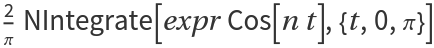.
Different choices for the definition of the Fourier cosine series expansion can be specified using the option FourierParameters.
With the setting FourierParameters{a,b}, the nth coefficient computed by ResourceFunction["NFourierCosCoefficient"] is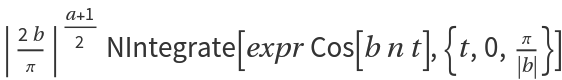.
The parameter b in the setting FourierParameters{a,b} must be numeric.
In addition to the option FourierParameters, ResourceFunction["NFourierCosCoefficient"] can also accept the options available to NIntegrate. These options are passed directly to NIntegrate.

## Examples

### Basic Examples (3)

Calculate a numerical approximation to a Fourier cosine coefficient:

 In:=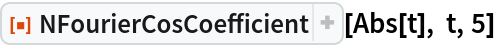Out=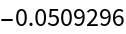Compare with the answer from symbolic evaluation:

 In:=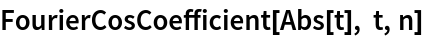Out=In:=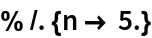Out=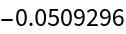Repeat the calculation, using a different definition for the Fourier cosine transform:

 In:=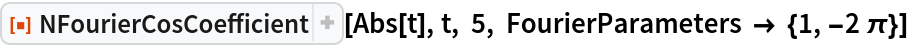Out=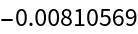## Requirements

Wolfram Language 11.3 (March 2018) or above

## Version History

• 1.0.0 – 26 April 2019